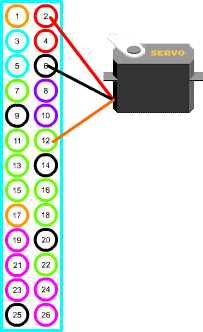# 型號規格# 接線

SG90的黑色線為接地, 需接到樹莓派實体編號的第6支接腳, 紅色線需輸入5V的電壓, 所以需接樹莓派的實体編號第2支接腳, 橘色線為控制線, 接GPIO 1之接腳# 極限值

SG90的脈衝寬大於一般的伺服馬達. 所以網路上有人說, 這樣子的話, 角度會大於180度, 但也有人說大於180度是錯誤的.

# 程式

package servotest;
import com.pi4j.wiringpi.Gpio;
import com.pi4j.wiringpi.GpioInterrupt;
static boolean closeFlag=false;
public static void main(String[] args){
int start=54;
int end=280;
int init=start;
Gpio.wiringPiSetup();
Gpio.pinMode(1, Gpio.PWM_OUTPUT);
Gpio.pwmSetMode(Gpio.PWM_MODE_MS);
Gpio.pwmSetRange(2000);
Gpio.pwmSetClock(192);
int angle=init;
int dir=1;
int speed=1;
int interval=100;
while(true){
Gpio.pwmWrite(1, angle);
try {Thread.sleep(interval);} catch (InterruptedException ex) {}
angle+=dir * speed;
System.out.printf(String.format("angle= %d\n", angle));
if(angle>=end || angle<=start)dir=-1*dir;
}
});
Gpio.pinMode(0, Gpio.INPUT);
System.out.println("Raspberry Pi PIN [" + event.getPin() +"] is in STATE [" + event.getState() + "]");
switch(event.getPin()){
case 0:
closeFlag=true;